# S13E2 - Equilibrium Constant Expressions, and How to Calculate Kc and Kp

## Equilibrium Constant Expressions

For the general reaction:

jA  +  kB  ⇌  ℓC  +  mD

An expression for chemical equilibrium can be written:As we can see above, the equilibrium constant (K) is equal to:

K = [C][D]m / [A]j[B]k  , where...

K (or K) is unitless and the [brackets] represent the concentrations at equilibrium.

==========

## How to Calculate the Equilibrium Constant, Kc

Now that we know how to write an equilibrium expression, how exactly do we calculate the equilibrium constant, K ?

First, there's 2 NOTES I want to make.

Then, we'll do a detailed example 😊

----------

➞ NOTE #1:  If the original reaction above is written in reverse, then we have:

K' = [A]j[B]k / [C][D]m  =  1 / K

Or, in a more visually appealing view...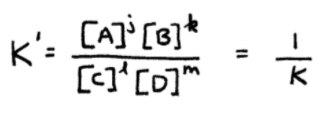----------

➞ NOTE #2:  If the original reaction at the beginning of this post is multiplied by a coefficient (i.e. 4), then for...

4jA  +  4kB  ⇌  4ℓC  +  4mD

We have:

K'' = [C]4ℓ[D]4m / [A]4j[B]4k  =  (K)4  =  K4

Or, in a more visually appealing view...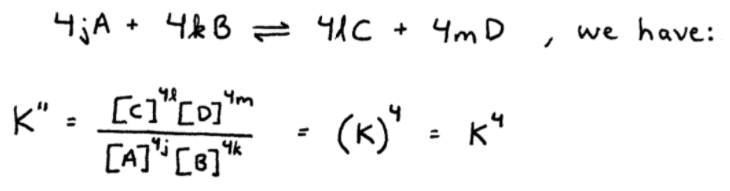==========

## Equilibrium Constant Calculations

Now, as promised, let's do some equilibrium constant calculation examples...

----------

ex:  Consider the following reaction and the following equilibrium concentrations: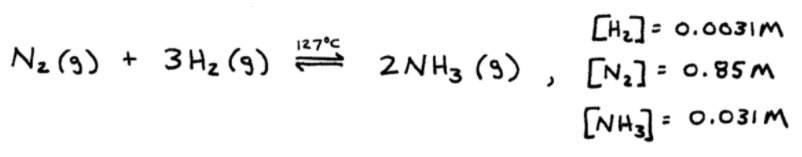a. Calculate the equilibrium constant, K.b.  Calculate K for the the reverse reaction.c. Calculate the equilibrium constant for the reaction:----------

So far today, we've seen how the value for K changes as we also change the reaction (reversing it, multiplying it through by 1/4th, etc).

However...

==========

## Equilibrium Positions

➞ There's only one equilibrium constant (K) for a specific reaction at a particular temperature...

But, there's an infinite number of equilibrium positions.

----------

Equlibrium Position  =  a set of equilibrium concentrations.

----------

ex:  Consider the reaction below, where 4 separate experiments are run

-- each with a different amount of initial concentrations of reactants and product.

N2(g)  +  3H2(g)  ⇌  2NH3(g)

_________

➞  as you can see in the image below, all 4 experiments (at the same temperature of course), give rise to one equilibrium constant...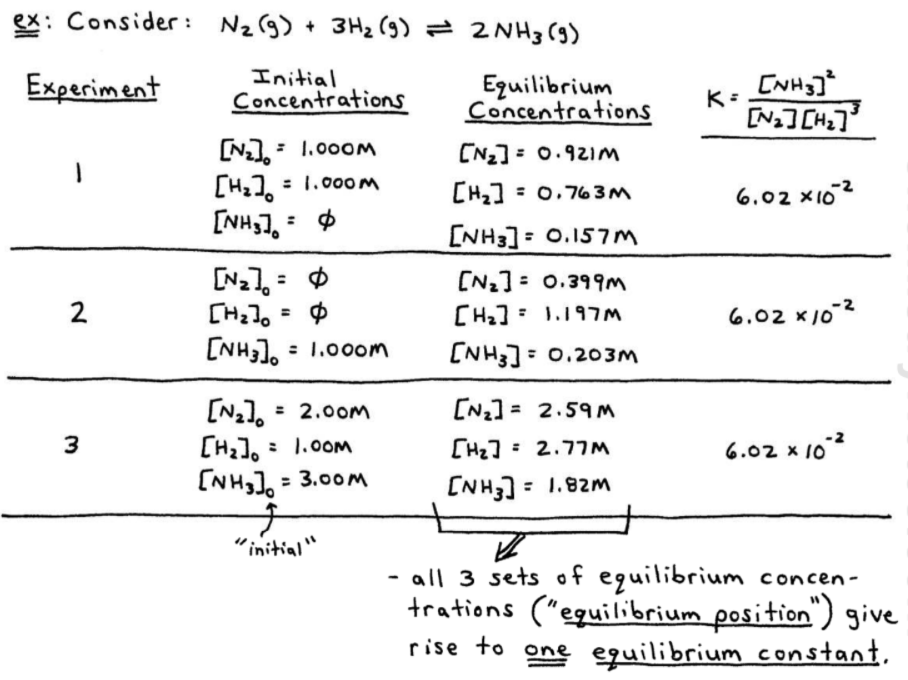All three sets of equilibrium concentrations, known as equilibrium positions, give rise to one equilibrium constant (K).

==========

## Equilibrium Partial Pressures and Kp

Besides being described via equilibrium concentrations, equilibria involving gases can also be described in terms of Equilibrium Partial Pressures, (instead of concentrations).

WHY ?

Because we can manipulate the Ideal Gas Law...

➞ Concentration (C) is moles / L , right?

➞ And so is "n / V" in the Ideal Gas Law (moles / L).

See here: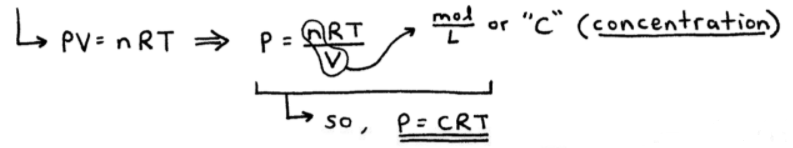Both R (the ideal gas constant) and T (temperature) are constant, so for the reaction,

N2(g)  +  3H2(g)  ⇌  2NH3(g)

We can represent equilibrium with either K (~ Kc) or Kp ...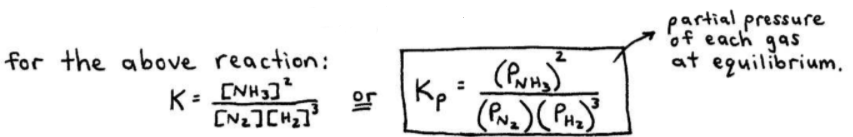----------

ex:  Calculate the value of Kp for the following reaction at 25°C given these equilibrium partial pressures:

PNOCl  =  1.2 atm
PNO  =  0.05 atm
PCl2  =  0.30 atm

2NO(g)  +  Cl2(g)   ⇌   2NOCl(g)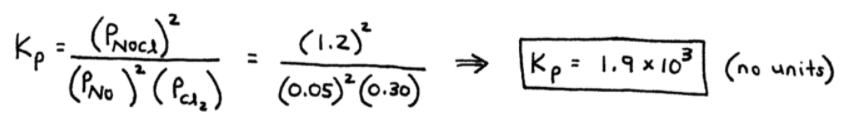==========

## Kc and Kp

The relationship between Kc and Kp is shown below:

R  =  universal gas constant (0.0821 L.atm / K.mol),
T  =  Kelvin temperature, and
Δn  =  the change in moles of gas particles (products − reactants).

### Kp  =  Kc(RT)Δn

==========

Stick around for the 3rd set of video notes on SECTION 13 - Chemical Equilibrium where we'll discuss,

Heterogeneous Equilibria.